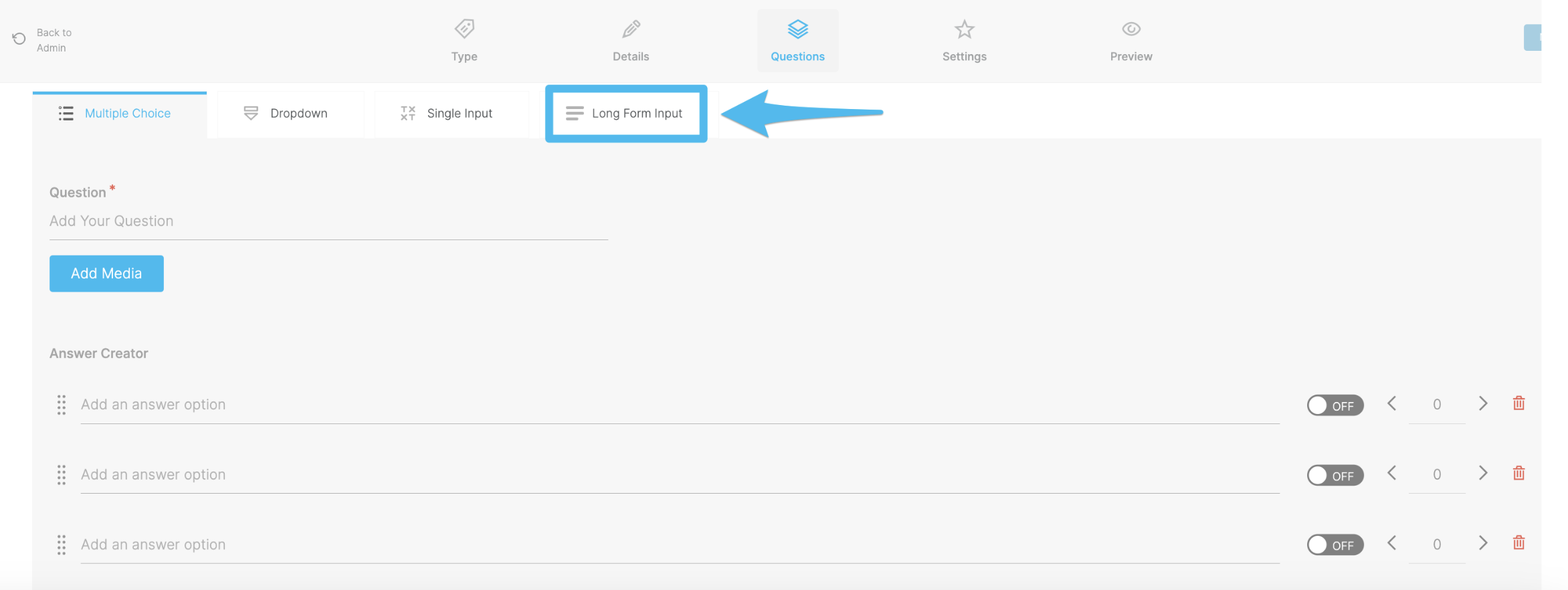# Text Question Types in Continu Follow

Multiple Choice Questions

Dropdown Questions

Single Input Questions## Multiple Choice Question

Complete the Question and the Answers that the learner can choose from:Indicate the correct answer by turning the switch beside the answer to OnThe point value for correct answers is automatically set to 1. You can manually change this value if you would like to have some questions worth more or fewer points than others.You can set further question integrity by selecting the Randomize Questions option. (This will ensure that each time a learner reviews the questions, the possible answers will always display in a random order).Turn a multiple-choice question into a multi-answer question, by turning On more than one answer valueSet a point value for the correct answer. (You may want to consider adding a lower value for this answer, to weigh the scores)Select the Allow Multiple Inputs optionChoose the number of answers you will accept for this questionSelect the Maximum Answer Value (optional), to allow the learner to receive the maximum points for each correct answer. This will automatically calculate the value, as indicatedSet a optional Custom Value, by selecting this optionSet the Custom Value## Dropdown Question

Complete the Question and the Answers that the learner can choose from:Indicate the correct answer by turning the switch beside the answer to OnSet a point value for the correct answerYou can set further question integrity by selecting the Randomize Questions option. (This will ensure that each time a learner reviews the questions, the possible answers will always display in a random order).## Single Input Question

Complete the Question and the Answers that the learner can choose from:For Single Input, you have the option to either have this question graded manually or by providing the correct answer and selecting the point valueSelect Allow Multiple Inputs, to allow a learner to provide more than one answer. (If this option is selected, ensure that you accurately score the correct answers)If the option to Allow Multiple Inputs is selected, you can set a Maximum Answer Value by selecting the optionSelect the Custom Value option, to set a specified point value for this question## Long Form Question

Complete the QuestionSet the Point Value for the questionSet the Word Minimum and Word Limit accordingly